Misprint Sensing System

(diff) ← Older revision | Latest revision (diff) | Newer revision → (diff)

This page describes the system that we are using in our misprint detection project.

 Document Sensing System

 Sensor characterization

For the current implementation, we use a CCD sensor that we could characterize last quarter in the laboratory. Here is the current used sensor.

Last quarter, we characterized the CCD sensor by evaluating its different noise parameters and its quantum efficiency curve. Here is the example of the quantum efficiency curve that we are currently using in the implementation. Then, we see that this sensor might easily be mistaken for some colors near blue and purple, as its sensitivity is pretty low. As a matter of fact, even in those colors, the final algorithm is still able to detect a missing cyan color.

Here is also a summary of the different noise characteristics of the sensor. For the current implementation, we have the possibility to use those characteristics, or to turn off the noise. Here is a summary of the noise characteristics obtained by experimentation in the laboratory.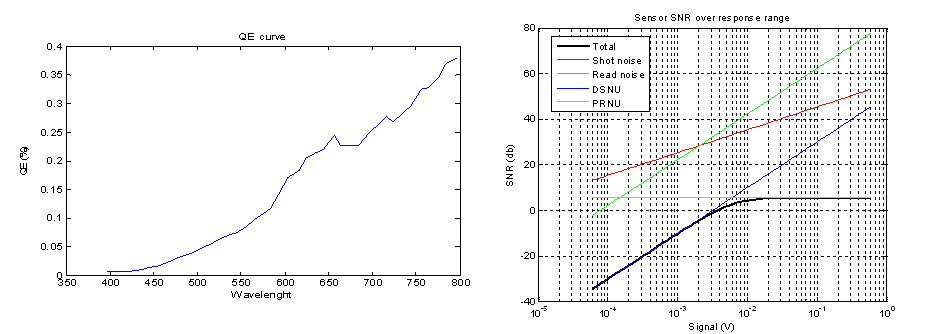Noise characteristics and QE curve

With this sensor model combined with a simple optical one, we have a good model of the transformation operated on an original light coming from the printing paper.

 From CMYK to reflectance

</td> </td>

The next part of the model is to be able to describe the reflectance of the printed paper given our original CMYK representation. For this, we used data provided by the HP labs giving us a serie of measurements for different printed papers. For a total of 1338 different CMYK values, we have 36 different measurements corresponding to 36 different wavelength and describing the reflectance property of the printed paper.

With those data, we could create two models describing the transformation between CMYK and reflectance. The first one use a nearest neighbors approach. Given a CMYK value we would like to transform, we find the closest neighbors from the table provided and average them to have an estimate of the reflectance that the paper would have for this particular CMYK value. This is a precise approach because the table provides a lot of data so averaging will not lead to a large error. The problem with this approach is the computational complexity. As the table is pretty large, finding the closest neighbors can take a lot of time when required for thousands of different CMYK value.

The fastest but less precise approach that we are currently using is based on a global model learnt from the table. We consider that, based on the Beer-Lambert law, the reflectance should depend as a logarithm of the density of the ink. Then, for each of the 36 different wavelengths, we tried a global log-linear model describing the reflectance for each wavelength of a log-linear combination of the CMYK coefficients. Using this approach, we know have a simple matrix containing much less coefficients than the original table and allowing a much faster conversion.

You can see on the following images the difference obtained by the two different approaches.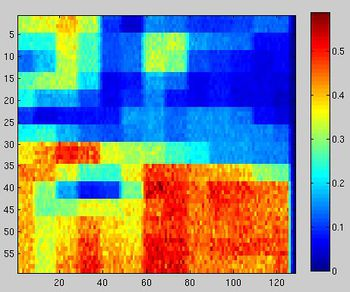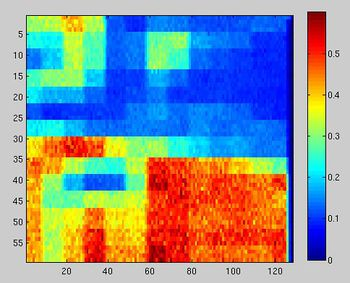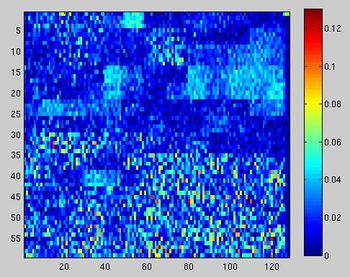Image from the slow mode Image from the fast mode Difference between these 2 images

Difference between the 2 images: the difference is within the noise level mostly.

 Simulating the 1D sensor

The original version of the algorithm considered the scene as a 2D reflectance version of our original image. This image could then be easily converted using our previous sensor and optimal model by simply adding a light and considering our sensor to be large enough to be able to observe the entire scene. To create a more realistic model that uses our one-line sensor, we developed a new strategy to convert our original image into a sensor response. We cut our original image in several smaller ones with same width, but containing only a small number of lines from the original image. Those smaller images can be considered as the scene our sensor would effectively observe at a given time. From each of those new scenes, we can know apply our one-line sensor model to obtain a one-line response. The combination of the different line responses obtained from the subimages now represent more accurately the way the sensor operates and visualizes the printed paper.

The only parameter that we had to decide was the light -as the ink properties were given- and as the sensor properties are defined by the sensor. There are 2 possibilities there:

• We can have a changing light so we always have the same luminance. The advantage is that we tend to stay in the low noise and no-saturation values of the sensor, but the output values for different CMYK patches become very close. And to distinguish them, we can only use the difference in the QE curve of the sensor for the different colors.
• Or we can have a fix light, this gives a much wider range of values for the sensor output and also makes much more simple the computation and the use of the look-up tables (see next paragraph). We can choose the light power in a way that we are at the saturation limit for the white, and so only the dimmest CMYK are in the very noisy area. This is the method we chose for these 2 reasons. We can check the choice of the light power on the following curve:

For a cmyk value of (i-1)*ones(1,1,4), we can find the output is varying in a reasonable fashion:

</td> </td>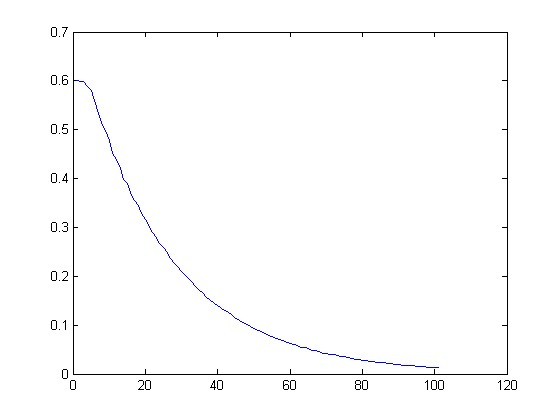Horizontal axis = i (ranging from 1 to 100) Vertical axis = Sensor output for the patch i*(1,1,1,1)

Finally this gives us the following result on an example:

</td> </td>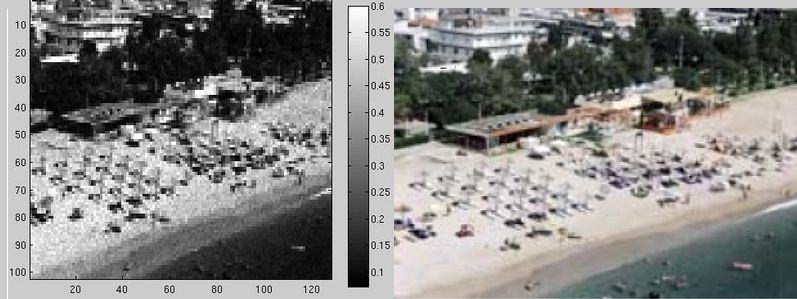Original and sensed image

 Using ISET, so we don't use it

With this whole simulation pipeline set up in ISET, we can simulate the response of our sensor for any image that we can describe in the CMYK space. I particular, we can simulate the response of the sensor for a completely uniform patch. This allows us to sample the CMYK space and to compute for each of these sample the sensor output. We can then build a look-up table for the whole simulation pipeline by running the simulator that we have in ISET on the different patches.

More precisely, by running ISET on a uniform patch, we can extract 2 data that we need to reconstruct a signal.

• The average value of the signal for the given CMYK.
• The variance of the deviation from this average.

We are doing several assumptions with this model:

1. We are assuming that this difference is a gaussian random variable, and so we can easily simulate this difference within MATLAB. Experiments show that it is a reasonable assumption.
2. The second assumption that we are doing is that there is no blur from the optics. Therefore, we can just split the image in sub-images of n lines. (For instance in pieces of 4 lines) and these n lines directly give the input to the sensor.
3. The last assumption is that compute an input sample of the sensor by averaging the CMYK values of the nxn square that corresponds to 1 pixel of the

sensor. This is clearly less true than averaging the ouput. But it reduces the computation by a factor of n².

It is important to see that these assumption give some deviation from the original model, but they are not limitations of the method, indeed, by paying more accuracy with some more computations, we can in each case having a more accurate result:

1. It is possible to describe, with a very good precision, the distribution of probability of the difference from the average. It just needs more simulations, and then a little more computation to generate it.
2. It is possible to simulate the blur from the optics by first converting all the CMYK samples in output (see next point) and then applying a convolution to simulate the blur from the optic.
3. We can find an output from each sample, and then average these outputs. Of course we then need to use the look-up table n² times more.

Finally, this method gives us a much faster way to simulate the sensor (between 60 and a 100 times faster). And even if some assumption don't make it completely accurate, the more important is that it gives us a realist simulator that we can use to test our misprint detector algorithm.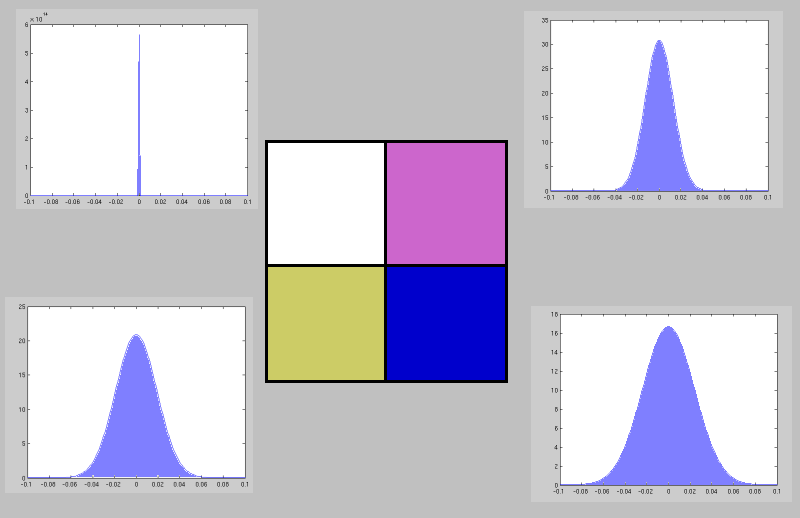Examples of difference noise distributions for pixels of different colors